# Basic Multiplication (0-10)

Times up!
Times up! You may finish answering the current question and then proceed to your score!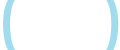Sorry, you have used all of your available hints for this lesson.
Practice Limit Reached

You've reached your daily practice limit of 12 questions.

Award InformationAwarded toQuestion Hint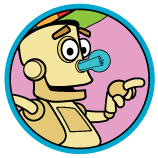Choose Family Member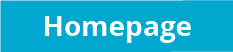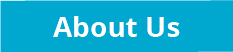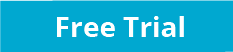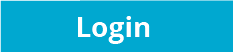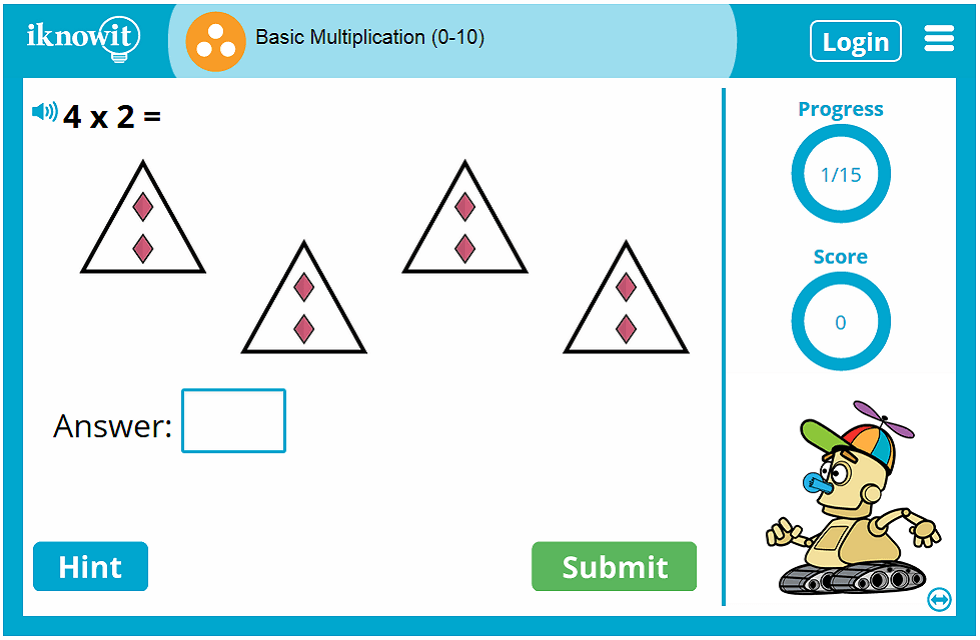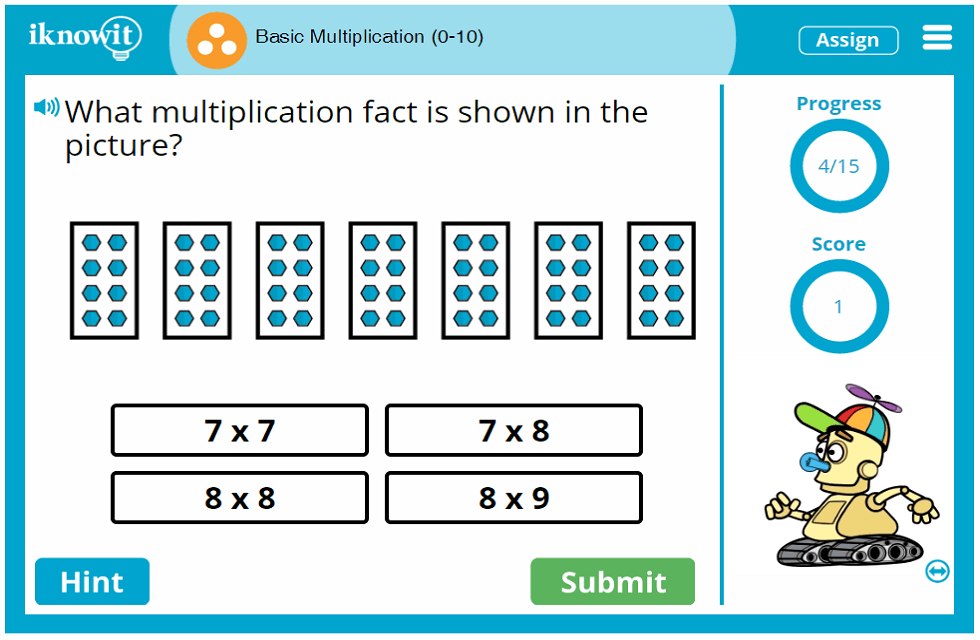## Interactive Math Game - Basic Multiplication (0-10)

Are you ready to practice basic multiplication from zero to ten? Let's dive into this third grade 'I Know It' lesson! Children will practice basic multiplication facts with factors ranging from 0 to 10. The problems will be presented to them in a variety of formats, including multiple choice, word problems, and fill-in-the-blank.

If you're curious about what kinds of problems your students will come across in this math lesson, here are some examples: “What multiplication fact is shown in the picture?” Choose from: 3 x 2, 1 x 2, 1 x 1, and 3 x 1. “Mr. Cantu has five packs of pencils. Each pack has eight pencils. How many pencils does Mr. Cantu have?” “5 x 1 =” (The basic multiplication facts are accompanied by a multiplication array.)

If students choose to use the hint option, they will be given a written clue, such as “1 group of 4” or “4 + 4 + 4 =”. If students answer the questions in this multiplication lesson incorrectly, the explanation page will show them the correct answer in written and pictorial form.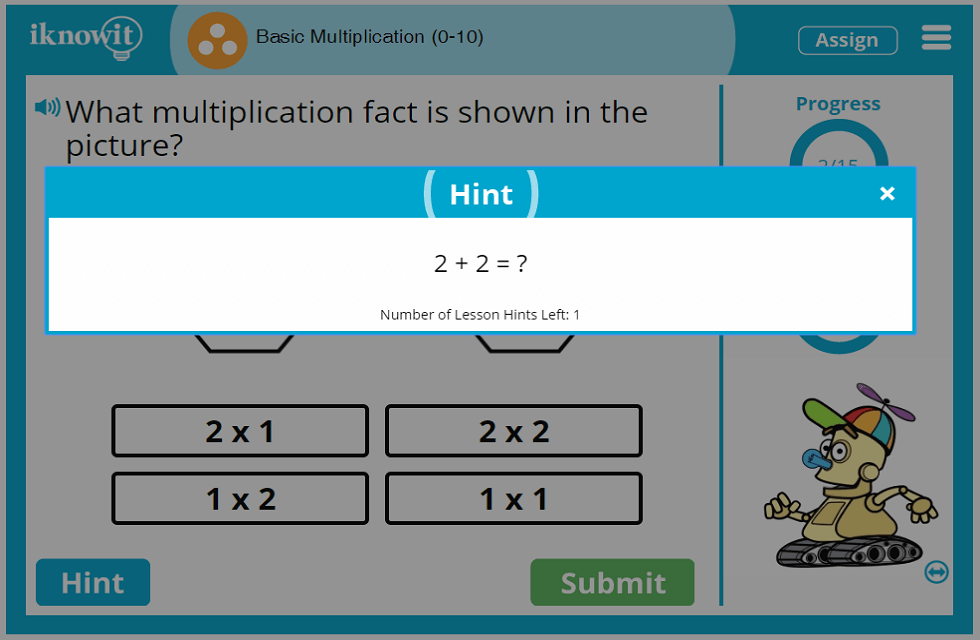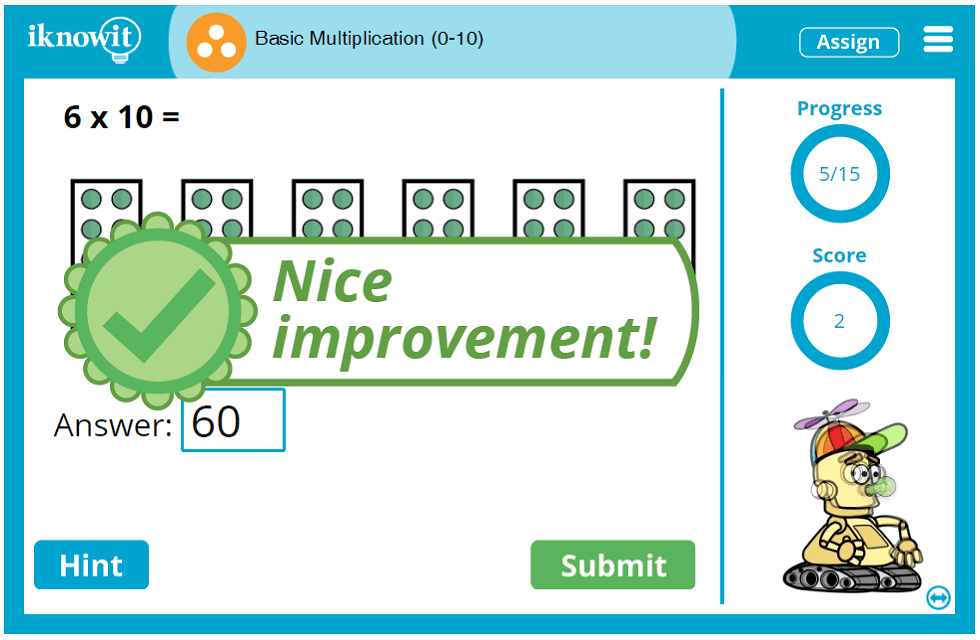## What Makes 'I Know It' Different?

'I Know It' is a math practice website geared toward elementary-aged students. You can use our online math program as a supplementary resource to your own math curriculum. Many teachers assign 'I Know It' lessons for extra math practice in the classroom or at home. You'll find that the kid-friendly design of our website, its easy-to-navigate layout, and bright, whimsical colors and characters appeal to students and teachers alike.

Interactive math games from 'I Know It,' including this basic multiplication lesson with numbers 0 through 10, include several features that help children make the most out of their math practice sessions. For example, the score-tracker on the right side of the screen helps students keep track of how many problems they've answered correctly and the progress-tracker lets them know how many more problems to go before they reach the end of the lesson. A 'hint' button provides a pictorial or written clue to help students solve the problem. Should they answer incorrectly, a detailed explanation page appears, showing them how they should have solved the problem, step by step.

Another important feature of all 'I Know It' math lessons is the speaker icon in the upper-left corner of the screen. Students can click this icon to have the math problem read aloud to them in a clear voice. What a great resource for ESL/ELL students and students who prefer auditory learning!

We hope this interactive multiplication lesson will help your students sharpen their basic multiplication skills. Be sure to try out this online math game and browse our other math topics!

## Free Trial and Membership Information

Your students will be able to try out this basic multiplication (0-10) lesson at no cost when you sign up for a free sixty day trial on iKnowIt.com. Students will be able to answer 25 total questions per day across all math lessons on 'I Know It'. For unlimited access to all math lessons, features, and administrative tools, you will need become a member of the site.

Your 'I Know It' membership will unlock all the features and administrative tools that make using our math program a pleasure! For example, you will be able to create a class roster and add your students, assign individual student login information, assign specific lessons to individual students, monitor student progress, download, print, and email student progress reports, and change basic lesson settings, such as limiting the use of hints during your students' math practice sessions.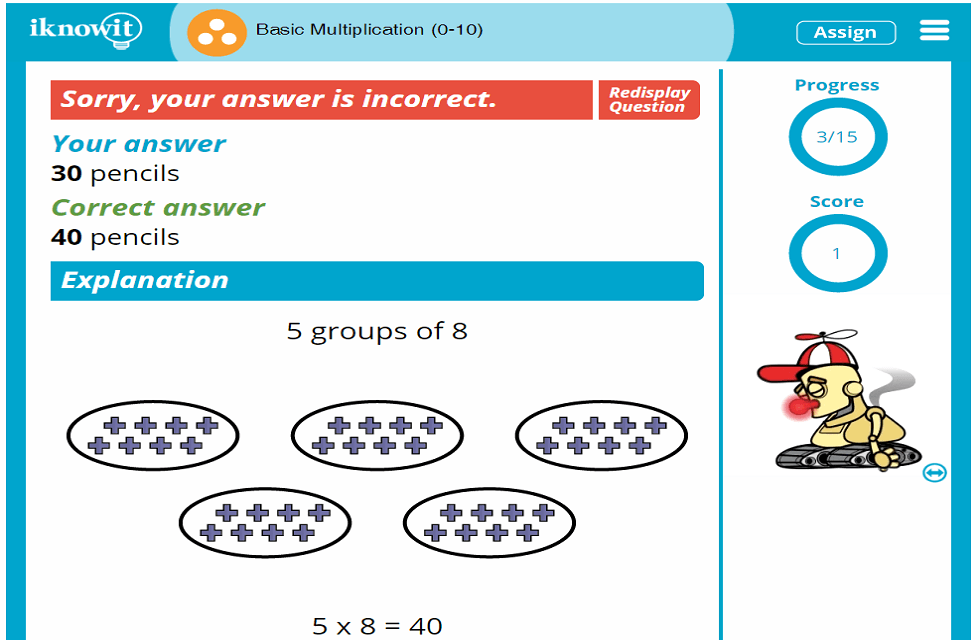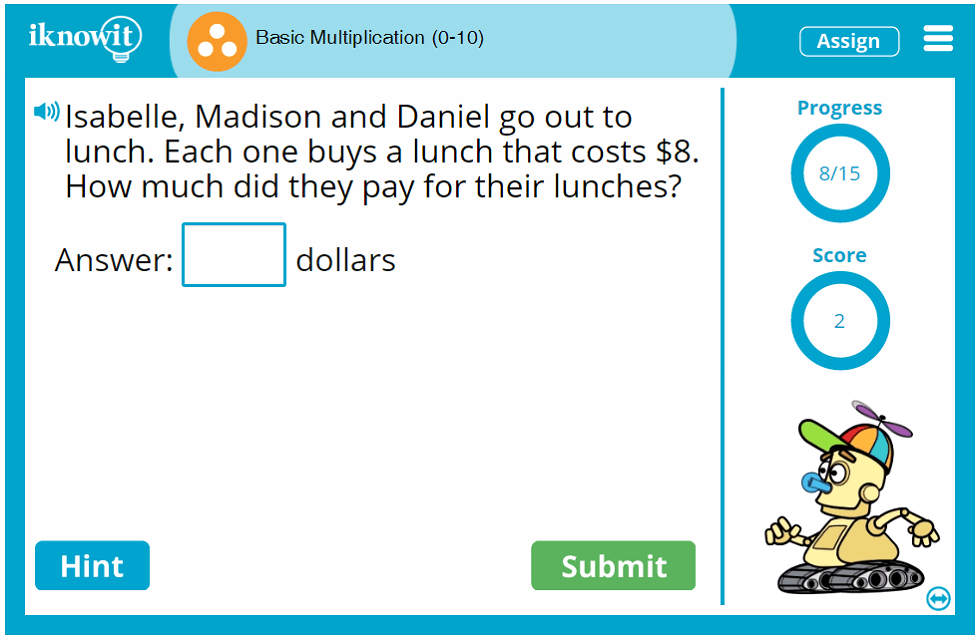## Level

This interactive math lesson on basic multiplication from zero through ten is categorized as a Level C lesson. You may want to give it a try with your third grade class.

## Common Core Standard

3.OA.7
Operations and Algebraic Thinking

Students will multiply and divide within 100, using strategies such as the relationship between multiplication and division or properties of operations. By the end of Grade 3, students will know from memory all products of two one-digit numbers.

## You might also be interested in...

Multiplication with Arrays (Level C)
In this third grade-level math lesson, students will practice multiplication using arrays. Students will solve multiple choice problems, fill-in-the-blank problems, and word problems.

Basic Multiplication (0-5) (Level C)
Students will practice basic multiplication facts in this third grade-level math lesson. Students will solve multiple choice questions, word problems, and fill-in-the-blank questions.